## Performing Partially Coherent Image Analysis

Category: Product News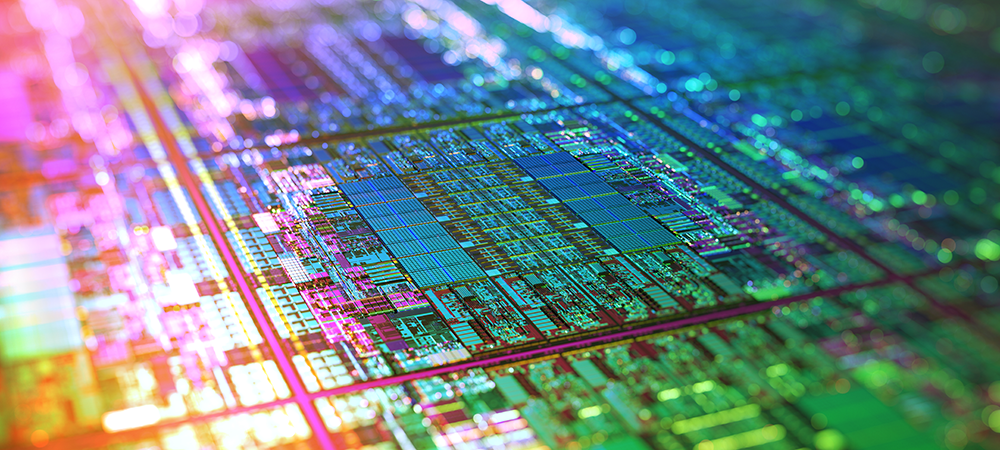In certain classes of optics, such as photolithography, or other small field of view, diffraction limited systems, coherence effects can have a large impact on image quality. The Partially Coherent Image Analysis in OpticStudio provides a powerful tool for simulating and analysing these effects. In this blog post we’ll provide an overview of the computations and discuss what to watch out for when performing partially coherent analysis.

Partially Coherent Image Analysis can be performed using any of three types of transfer functions: incoherent, coherent and partially coherent.

A partially coherent transfer function is more complex than incoherent or coherent because each point on the source will have a different level of coherence with respect to each other source point. The degree of coherence between points is dependent upon a parametric function. In general, the closer two points are, the more coherent that they will be.

Partially coherent transfer functions are appropriate for many real-world sources. For example, in photolithographic applications, the typical sources used are not fully coherent. The effect of partial coherence on the imaging of a mark onto a wafer is very important.

### Defining the degree of partial coherence

The image below shows a Cooke triplet photographic objective setup to work with on-axis light.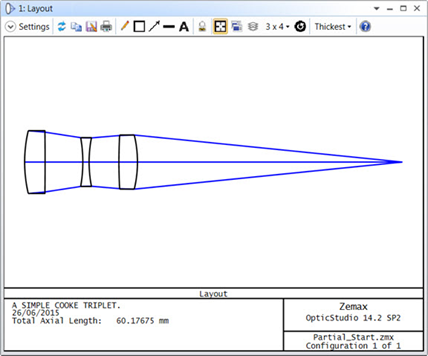In OpticStudio, partially coherent transfer functions are characterized using a parametric function, Gamma. The Gamma function utilized for a particular analysis can be one of two types, Gaussian or Sinc: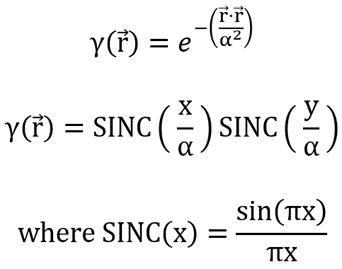For the Gaussian Gamma function, the position vector, r, represents the distance between two points in the displayed image.

For both functions, the parameter α (alpha) is a scaling parameter defined in lens units. This parameter sets the effective width of the Gamma function. The smaller that alpha is, the narrower the resulting Gamma function. Narrow gamma functions produce partially coherent images that are, for the most part, incoherent.

On the other hand, larger alpha values produce comparatively broad gamma functions. Broad Gamma functions generate partially coherent images that are very similar to coherent images.

In the settings of Analyze...Extended Scene Analysis...Partially Coherent Image Analysis. We can choose to visualise the Gamma function directly by setting “Data Type” to “Partially Coherent Test: Gamma”. The resulting Gamma function for an "Alpha" value of 0.0025 is relatively narrow and will produce partially coherent images that are mostly incoherent: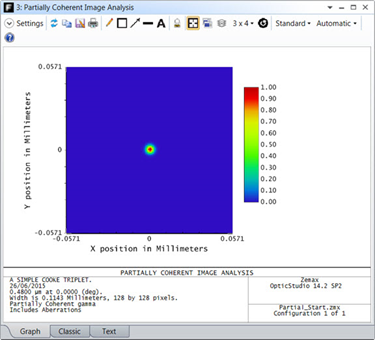Changing “Alpha” to 0.1 results in a Gamma function that is very broad and will produce partially coherent images that are mostly coherent: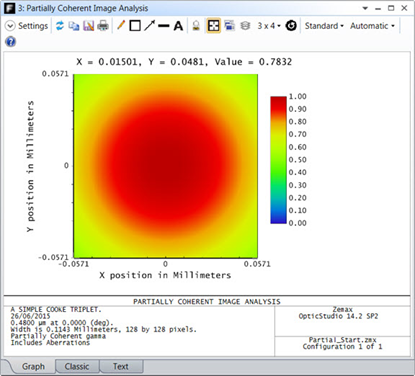### Computation methods for partially coherent transfer functions

When using partially coherent transfer functions for Partially Coherent Image Analysis, there are two computation methods available, Mostly Coherent and Mostly Incoherent. The method that you should use depends upon how broad the Gamma function that you are using is.

Narrow Gamma functions (i.e. Gamma functions that comprise less than 20% of the overall image) are narrow in the spatial domain and, thus, broad in the spatial frequency domain. As such, for these types of Gamma functions, it is more efficient to compute the Partially Coherent Image Analysis in the spatial domain. The Mostly Incoherent computation algorithm is designed for these narrow Gamma functions and, as a result, computations performed using this method are done in the spatial domain. On the other hand, broad Gamma functions (i.e. Gamma functions that comprise more than 20% of the overall image) are broad in the spatial domain and, hence, narrow in the spatial frequency domain. Thus, for these types of Gamma functions, it is more efficient to compute the analysis in the spatial frequency domain. The Mostly Coherent algorithm is designed for these broad Gamma functions. For this algorithm, computations are performed in the more efficient spatial frequency domain.

If you try to use the Mostly Incoherent method for a broad Gamma function (or, conversely, the Mostly Coherent method for a narrow Gamma function), the computation will take significantly longer at best. Even worse, the computation may not be carried through to completion due to insufficient sampling. So, it is always important to visualize the Gamma function that you are using, as described on the previous page, to determine which computation method you should use. In summary: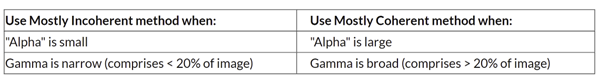There is one more related parameter of interest, the “Fraction” parameter. Both the Mostly Incoherent and Mostly Coherent methods speed up the Partially Coherent Image Analysis computations by making the assumption that the Gamma function has a finite width. (In reality, Gamma and Sinc functions have infinite extent.) You have control over this width via the “Fraction” setting. This parameter sets the fraction of total energy within Gamma that should be considered. In general, “Fraction” values larger than 0.96 will significantly slow down Partially Coherent Image Analysis computations given the asymptotic nature of Gaussian and Sinc functions.

### Performing incoherent/coherent analysis

Changing the “Data Type” to Raw Image (no diffraction) displays the source that we are going to image through the Cooke triplet, a three bar target. It is important to keep in mind that, in principle, any IMA file could be used: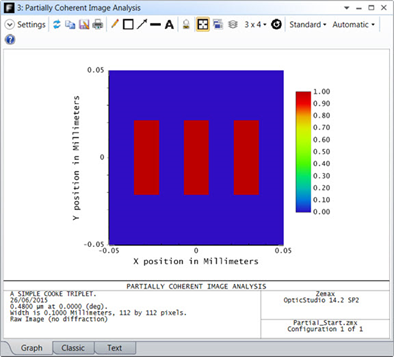Next, we are going to take a look at two extremes, a fully incoherent image of this source and then a fully coherent image. First, we generate a perfectly incoherent image by changing “Data Type” to Incoherent Image. Notice that the resulting image shows the general blurring expected (as a result of diffraction) but no coherent interference:Next, we change “Data Type” to Coherent Image. Observe that the image now displays clear evidence of spatial interference, especially at the corners of the bars: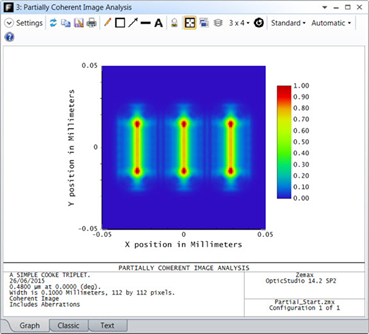### Performing partially coherent analysis

Now, it is time to look at the partially coherent results. First, let us try a small "Alpha" value (i.e. 0.0025). We know that for small "Alpha" values, the resulting Gamma function is narrow and, as such, the results are mostly incoherent. With “Data Type” set to Partially Coherent Image (Mostly Incoherent Method) and “Alpha” of 0.0025 the resulting image looks very much like the incoherent one we recently saw. The narrower the Gamma function, the more incoherent the resulting image will appear to be: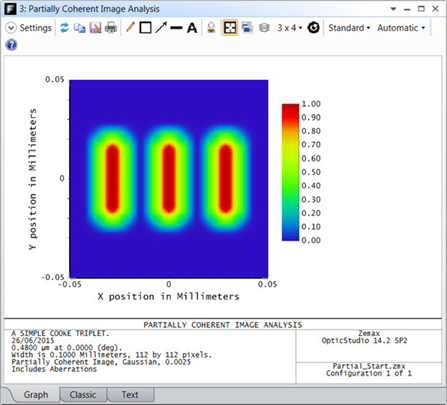Now, let us try a large "Alpha" value of 0.1. We know that for large "Alpha" values, the resulting Gamma function is broad. As a result, the results are mostly coherent. In this case we use “Data Type” equals Partially Coherent Image (Mostly Coherent Method). Notice that, not surprisingly, the resulting image looks very much like the coherent result we saw previously. The broader the Gamma function, the more coherent the resulting image will appear to be:Now, we’ll try an "Alpha" value that is neither small nor large (i.e. 0.01). First, let’s take a look at the Gamma function. The Partially Coherent Test:  Gamma displays Gamma function that is narrower than the function for an "Alpha" value of 0.1 but more broad than that for an "Alpha" of 0.0025, as expected: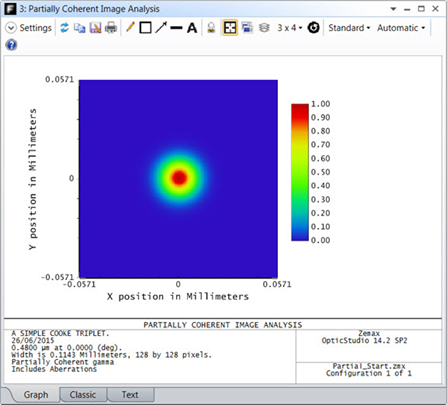From the display, it is clear that the Gamma function covers more than 20% of our image, so we will still use the Mostly Coherent method here. The resulting image clearly shows spatial interference effects, but, as expected, these effects are not as pronounced as they are for a broader Gamma function as we just saw (i.e. "Alpha" = 0.1):### Sampling issues

It is important to note that the image must be sufficiently sampled to generate accurate partial coherence results. To verify that the sampling is sufficient, you can look at the PSF that is being used in the partially coherent calculations. With “Data Type” set to Partially Coherent Test:  PSF, we Zoom-in on the non-zero portion of the PSF: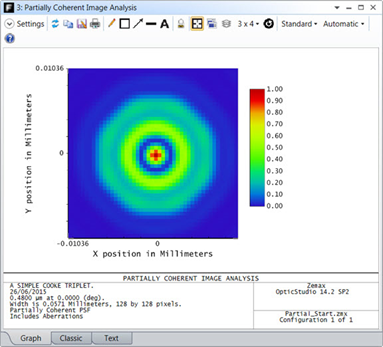Adequate sampling is achieved when there are at least 10 points displayed over the non-zero portion of the PSF, which is the case here. If the sampling is not adequate, try increasing the “Oversampling”. In general, if the sampling is not sufficient, OpticStudio will issue a “Sampling Too Low, Data Inaccurate!” message when you try to generate the PSF or partially coherent image: